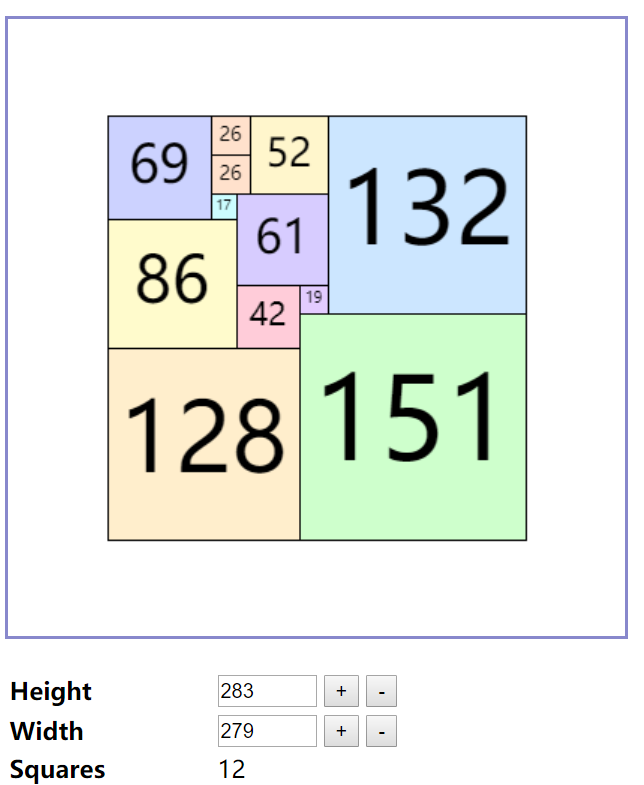🏆 第 160 场力扣周赛3 分 - 找出给定方程的正整数解
5 分 - 循环码排列
5 分 - 串联字符串的最大长度
7 分 - 铺瓷砖

• 找出给定方程的正整数解
数据范围在 $[1,1000]$，所以 $O(n^2)$ 的暴力解可以过。但其实这题和 LeetCode 74 是一模一样的，是双指针的一道非常经典的题目。大家可以参考 $74$ 的题解，这里就不详说了。最优复杂度为 $O(n)$

• 循环码排列
用归纳法证明：

1. 首先，只有一位的时候，我们可以得到一个合法序列 $[0,1]$，我们用序列 $s$ 表示。
2. 现在来考虑两位的情况，我们在前面的序列中都加上 $0$$1$，可以得到 $[00,01]$$[10,11]$ 两个序列组，我们也称为序列 $left$ 和序列 $right$同一个序列组一定满足相邻只相差一位，因为他们的第一位补充的都是一样的。另外一个结论是， $left$ 序列的第一个串，和 $right$ 序列的第一个串是只相差一位的(只有我们补充的第一位不同)，同理，$left$ 序列的最后一个串，和 $right$ 序列的最后一个串是只相差一位的。那么，如果我们把 $right$ 序列转置和 $left$ 序列拼接，最后就能得到一个合法的序列排列
3. 同理，对于 $n$ 更大的情况，也可以类似的得到结果。

时间复杂度为 $O(n^2)$

• 串联字符串的最大长度
由于字符串数量只有 $16$ 个，我们可以枚举每个字符串用或者不用，来暴力所有的结果，时间复杂度为 $O(2^n*n*m)$$m$ 为字符串长度。可以用动态规划优化一下转移降低为 $O(2^n*m)$ 复杂度。

• 铺瓷砖
这题争议比较大，各种解法也很多，先上结论：这题是一个NP问题，所以所有多项式复杂度算法都存在问题。下面给一个例子，多项式解可以验证一下，更多的例子可以参考参考网站所以我们只需要关注搜索解法就可以了。有一种主流的写法是存储一个二维状态数组，记录每个格子是否被占用，然后每次取最左上的空格子，枚举放置的正方形长度来进入下一个状态。这种做法也能通过题目，但是可以稍微简化一下，我们可以只用一个一维数组记录状态，数组的每一个值是 这一行目前被占用的长度，比如 $[1,2,1]$，对应着：

[[1,0,0],
[1,1,0],
[1,0,0]]

然后我们每次枚举 当前长度最短的行，然后以这点作为要放置的正方形的左上角，枚举要放置的正方形的边长。理论上的状态总数为 $n^n$，每次枚举的方法数为 $n$ 种。 理论时间复杂度上界为 $O(n^{n+2})$

1